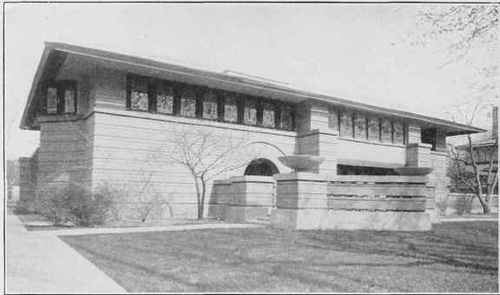Every solution for T-beam construction should be tested at least to the extent of knowing that there is no danger of failure on account of the shear between the beam and the slab, either on the horizontal plane at the lower edge of the slab, or in the two vertical planes along the two sides of the beam. Let us consider a T-beam such as is illustrated in Fig. 106. In the lower part of the figure is represented one-half of the length of the flange, which is considered to have been separated from the rib. Following the usual method of considering this as a free body in space, acted on by external forces and by such internal forces as are necessary to produce equilibrium, we find that it is acted on at the left end by the abutment reaction, which is a vertical force, and also by a vertical load on top. We may consider P' to represent the summation of all compressive forces acting on the flanges at the center of the beam. In order to produce equilibrium, there must be a shearing force acting on the under side of the flange. We represent this force by Sh. Since these two forces are the only horizontal forces, or forces with horizontal components, which are acting on this free body in space, P' must equal Sh. Let us consider z to represent the shearing force per unit of area. We know from the laws of mechanics, that, with a uniformly distributed load on the beam, the shearing force is maximum at the ends of the beam, and diminishes uniformly towards the center, where it is zero. Therefore the average value of the unit-shear for the half-length of the beam, must equal \z. As before, we represent the width of the rib by b. For convenience in future computations, we shall consider L to represent the length of the beam, measured in feet. All other dimensions are measured in inches. Therefore the total shearing force along the lower side of the flange, will be:Exterior View.Living Room.

RESIDENCE OF MR. ARTHUR HEURTLEY, FOREST AVENUE, OAK PARK, ILL.

Frank Lloyd Wright, Architect, Oak Park, 111.

Walls of Brick, Salmon Color; Roof of Shingles. Cost, \$14,000. A Strong and Unique Treatment of a Residence Design.Fig. 106. Analysis of Stresses in T-Beam.

Sh =1/2z X b X 1/2L X 12 =3bzL.(40)

There is also a possibility that a beam may fail in case the flange (or the slab) is too thin; but the slab is always reinforced by bars which are transverse to the beam, and the slab will be placed on both sides of the beam, giving two shearing surfaces.

## 294. Numerical Illustration

It is required to test the beam which was computed in Example 1 of Article 291. Here the total compressive stress in the flange =1/2 cbkd = 1/2 X 432 X 96 X 344 = 71,332 pounds. But this compressive stress measures the shearing stress Sh between the flange and the rib. This beam requires six 7/8-inch bars for the reinforcement. We shall assume that the rib is to be 11 inches wide, and that four of the bars are placed in the bottom row, and two bars about 2 inches above them. The effect of this will be to deepen the beam slightly, since d measures the depth of the beam to the center of the reinforcement, and, as already computed numerically in Article 290, the center of gravity of this combination will be 8/10 of an inch above the center of gravity of the lower row of bars. Substituting in Equation 40 the values Sh = 71,332, b = 11, and L = 20, we find, for the unit-value of z, 108 pounds per square inch. This shows that the assumed dimensions of the beam are satisfactory in this respect, since the true shearing stress permissible in concrete is higher than this.

But the beam must be tested also for its ability to withstand shear in vertical planes along the sides of the rib. Since the slab in this case is 5 inches thick and we can count on both surfaces to withstand the shear, we have a width of 10 inches to withstand the shear, as compared with the 11 inches on the underside of the slab. The unit-shear would therefore be 11/10 of the unit-shear on the under side of the slab, and would equal 119 pounds per square inch. Even this would not be unsafe, but the danger of failure in this respect is usually guarded against by the fact that the slab almost invariably contains bars which are inserted to reinforce the slab, and which have such an area that they will effectively prevent any shearing in this way.

Testing Example 2 similarly, we may find the total compression C from Equation 32, and that it equals As = 16,000 X 3.37 = 54,000 pounds. The steel reinforcement is six 3/4-inch bars, and by Table XVIII we find that if placed side by side, the beam must be 13.19 inches in width, or, in round numbers, 13 1/4 inches. Substituting these values in Equation 37, we find, for the value of z, 45 pounds per square inch. Such a value is of course perfectly safe. The shear along the sides of the beam will be considerably greater, since the slab is only four inches thick, and twice the thickness is but 8 inches; therefore the maximum unit-shear along the sides will equal 45 times the ratio of 13.25 to 8, or 75 pounds per square inch. Even this would be perfectly safe, to say nothing of the additional shearing strength afforded by the slab bars.